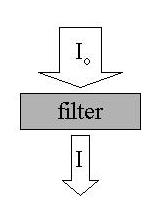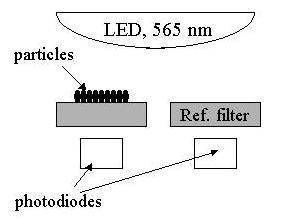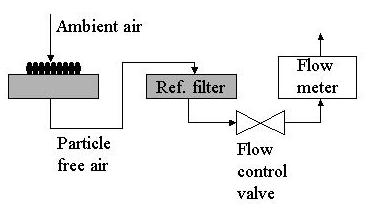### Description of Particle Soot Absorption Photometer (PSAP)

The PSAP is a filter-based method for determining light absorption.

#### General Information

The Particle Soot/Absorption Photometer (PSAP) is used to measure in near real time the light absorption coefficient. The method is based on the integrating plate technique in which the change in optical transmission of a filter caused by particle deposition on the filter is related to the light absorption coefficient of the deposited particles using Beers Law. Older versions operated at 1 wavelength (nominally 565 nm) while new versions have 3 wavelengths (467, 530 and 660 nm). The description below is for the 1 wavelength version, but the principle is the same for the 3 wavelength instrument.

Beers Law is given by:

A=ln(Io/I)where

• A is the absorbance - how much light was absorbed while passing through the filter
• I is the intensity of light transmitted
• Io is the original intensity of light before passing through the filter.

Calculation of absorption coefficient is based on the change in filter transmission (I/Io) for a given volume of sample air. Without correcting for filter type and loading, the absorption coefficient is:

bap = (area/volume)ln(Io/I)

where

• bap is the absorption coefficient [m-1]
• Area is the area of the sample spot [m2] - different for each instrument
• volume is the volume of air sampled in averaging period [m3]
• I is the average filter transmittance for averaging period, j+1
• Io is the average filter transmittance for averaging period, j.

With correction for filter nonlinearity (loading+filter characteristics?) the corrected absorption coefficient is:

bap,corr = bap f(Tr)

where f(Tr) is the transfer function based on filter loading (Tr) for Pallflex filters. The transfer function is incorporated in the software.

In order to ensure that the observed change in filter transmittance is not due to changes in the intensity of the LED light source, a second filter is used as a reference. This reference filter is adjacent to the primary filter.The particle laden airstream first passes through the primary filter which removes the particles and then the clean airstream passes through the reference filter.#### Corrections to PSAP measurements

We make several corrections to the PSAP measurements to obtain our final value for absorption coefficient. These corrections are described in detail Bond et al., (1999) but are briefly mentioned here. An update to these corrections for three-wavelength absorption measurements is provided in Ogren, 2010
• Spot size correction - each PSAP instrument has a slightly different spot area due to differences in machining of the the filter holder.
• Scattering aerosol correction - the aerosol collected on the filter will also scatter light which is observed as a lowering of transmittance of the filter.
• Transmittance threshholds - transmittances values below 0.5 are considered invalid, transmittance values below 0.7 are flagged as possibly suspect.
• The PSAP flow meter is calibrated with a primary flow standard
• The calibrations are referenced to 550 nm for consistency with the nephelometer green wavelength.

#### Measurement Uncertainties

These values are from Bond et al. (1999), and are reported at 95% confidence

• Flow rate calibration - typically less than 2%
• Spot size correction - typically less than 2%
• Response to scattering ~2%
• Response to absorption ~22%
• Instrument precision (unit-to-unit variability) < 6%
• Total estimated uncertainty of PSAP measurement at 60 s averaging time and typical atmospheric absorption levels ~15%

#### References

• Bond,T.C., Anderson, T.L., Campbell, D.,"Calibration and intercomparison of filter-based measurements of visible light absorption by aerosols," Aerosol Science and Technology, 30, 582-600, 1999.
• Ogren, J.A., "Comment on “Calibration and Intercomparison of Filter-Based Measurements of Visible Light Absorption by Aerosols,” Aerosol Science and Technology, 44:589–591, 2010.